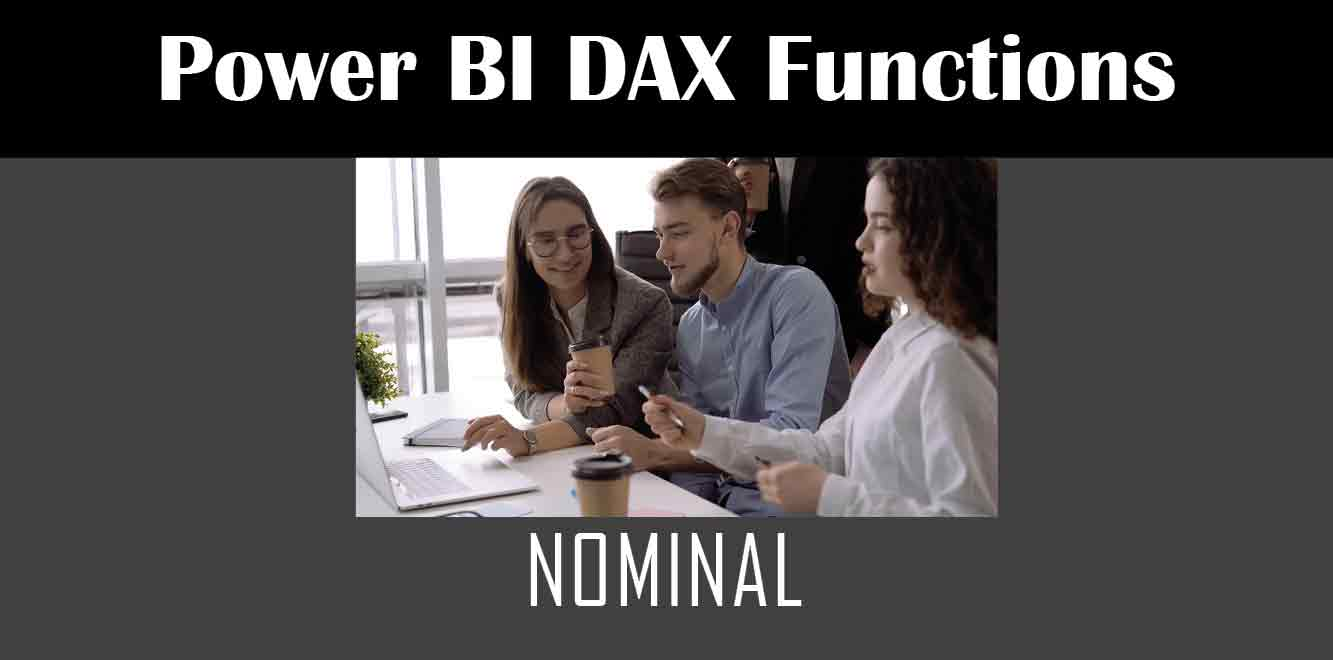# NOMINAL

## Y## What is the NOMINAL Function in Power BI?

The NOMINAL function in Power BI returns the nominal annual interest rate, given the effective rate and the number of compounding periods per year. The function is useful in financial analysis, especially when calculating the interest rate on loans or investments that have a compounding period.

## Syntax of the NOMINAL Function

The syntax of the NOMINAL function is as follows:

NOMINAL(effect_rate, npery)

``` The arguments of the function are: - `effect_rate`: The effective annual interest rate - `npery`: The number of compounding periods per year How to Use the NOMINAL Function in Power BI Now that we know the syntax of the NOMINAL function let's take a look at how we can use it in Power BI. Suppose we have a table consisting of a loan amount, an annual interest rate, and the number of payments per year. We want to calculate the nominal interest rate for each loan. Here is how we can do it: 1. Open Power BI Desktop and create a new report. 2. Import the loan data into Power BI using the "Get Data" option. 3. Once the data is loaded into Power BI, create a new measure by clicking on "New Measure" in the "Modeling" tab. 4. In the formula bar that appears, type in the following DAX formula: ```

Nominal_Interest_Rate = NOMINAL([Annual_Interest_Rate]/[Number_of_Payments_Per_Year], [Number_of_Payments_Per_Year])

``` 5. Press "Enter" to apply the formula, and the new measure will be created. 6. Now, drag and drop the "Loan Amount" and "Nominal_Interest_Rate" measures into a table or any visual of your choice. 7. You will see the nominal interest rate calculated for each loan. In conclusion, the NOMINAL function in Power BI is a useful tool to calculate the nominal interest rate for loans or investments that have compounding periods. By following the steps outlined above, you can easily use the NOMINAL function in Power BI to analyze your financial data. Power BI DAX Training Courses by G Com Solutions (0800 998 9248)Power BI DAX Intensive Training Course £1,050.00 – £26,550.00 Select optionsContinue Loading DonePower BI DAX Introduction £395.00 – £9,750.00 Select optionsContinue Loading DonePower BI DAX Intermediate £395.00 – £9,750.00 Select optionsContinue Loading DonePower BI DAX Advanced £395.00 – £9,750.00 Select optionsContinue Loading Done Upcoming Courses Contact Us Subject Your Name (required) Company/Organisation Email (required) Telephone Training Course(s) Power BI Intensive TrainingPower BI introduction Power BI IntermediatePower BI AdvancedDAXPower Query MPower BI CertificationPower BI AdministrationPower PlatformPower AutomatePower AppsOTHER Your Message Upload Example Document(s) (Zip multiple files) ```
``` ```
``` ```
``` ```
``` ```
``` ```